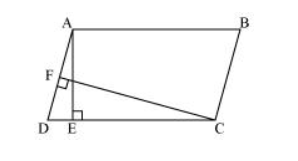# In the given figure, ABCD is parallelogramQuestion. In the given figure, $A B C D$ is parallelogram, $A E \perp D C$ and $C F \perp A D$. If $A B=16 \mathrm{~cm}, A E=8 \mathrm{~cm}$ and $C F=10 \mathrm{~cm}$, find $A D$.Solution:

In parallelogram $A B C D, C D=A B=16 \mathrm{~cm}$

[Opposite sides of a parallelogram are equal]

We know that

Area of a parallelogram $=$ Base $\times$ Corresponding altitude

Area of parallelogram $A B C D=C D \times A E=A D \times C F$

$16 \mathrm{~cm} \times 8 \mathrm{~cm}=\mathrm{AD} \times 10 \mathrm{~cm}$

$\mathrm{AD}=\frac{16 \times 8}{10} \mathrm{~cm}=12.8 \mathrm{~cm}$

Thus, the length of $A D$ is $12.8 \mathrm{~cm}$.